# Principal Component Analysis using Matlab

P

PCA is a way of identifying patterns in data, and expressing the data in such a way as to highlight their similarities and differences. Below are the steps of the algorithm:

```close all;

% clear all;

clc;

% data = data.data{1,2};

```

#### Step 1

– Initialize the dataset, 6 vectors of 32 sample data

```%
% Step 1 - Initialize the dataset, 6 vectors of 32 sample data
%

X = data(1:32,1:6);

```

#### Step 2

– Subtract the mean from each of the data dimensions. The mean subtracted is the average across each dimension.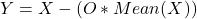, where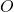is a vector of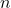ones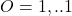```%
% Step 2 - Subtract the mean from each of the data dimensions.
%        - The mean subtracted is the average across each dimension.
%

Y = X - repmat(mean(X),size(X,1),1);

```

#### Step 3

– Calculate the covariance matrix of Y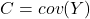```%
% Step 3 - Calculate the covariance matrix of Y
%

C = cov(Y);

```

#### Step 4

– Calculate the eigen(vect/vals) of the covariance matrix C.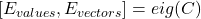```%
% Step 4 - Calculate the eigen(vect/vals) of the covariance matrix C.
%

[eigenvectors,lamda] = eig(C,'vector');

```

#### Step 5

– Sort the eigenvectors by eigenvalue, highest to lowest.

```%
% Step 5 - Sort the eigenvectors by eigenvalue, highest to lowest.
%

lamda = lamda(end:-1:1);

eigenvectors = eigenvectors(end:-1:1,:);

```

#### Step 6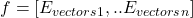where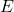are the eigenvectors and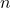depends on the level of compression.

```%
% Step 6 - Select eigenvectors and form a feature matrix.
%

percentance = 0;

until = 0;

while percentance < 97

percentance = sum(lamda(1:until+1)) * 100 / sum(lamda);

until=until+1;
end

features = eigenvectors(:,1:until);

```

#### Step 7

– Derive the new data set. We take the transpose of the vector and multiply it on the left of the transposed original data set.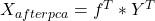```%
% Step 7 - Derive the new data set.
%        - We take the transpose of the vector and multiply it on the left
%        - of the transposed original data set.
%

Xn = (features' * Y')';

```

#### Step 8

– Get the original data back. We take the features vector and multiply it on the left of the transposed PCA produced data set Xn. This procuces the Y matrix. To get the actual X data then we have to add the mean of each vectors (see Step 2).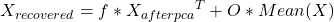.

```% Extra
% Step 8 - Get the original data back.
%        - We take the features vector and multiply it on the left
%        - of the transposed PCA produced data set Xn. This procuces the Y
%        matrix. To get the actual X data then we have to add the mean of
%        each vectors (see Step 2).
%

Xr = (features * Xn')' + repmat(mean(X),size(X,1),1);

```

#### Step 9

– Plotting.

```% Extra
% Step 9 - Plotting.
%

figure;
subplot(2,3,1);
plot(X);
ylim([-100 100]);
xlabel('X samples');
ylabel('vectors');
subplot(2,3,2);
bar(mean(X));
ylim([0 35]);
xlabel('vectors of X');
ylabel('Mean of each vector');
subplot(2,3,3);
bar([mean(mean(X)),mean(mean(Y))]);
ylim([0 20]);
xlabel('X Y');
ylabel('mean(mean(X)),mean(mean(Y))');
subplot(2,3,4);
plot(Y);
ylim([-100 100]);
ylim([-100 100]);
xlabel('Y samples');
ylabel('vectors');
subplot(2,3,5);
bar(mean(Y));
ylim([0 35]);
xlabel('vectors of Y');
ylabel('Mean of each vector (almost 0)');
ylim([0 35]);
subplot(2,3,6);
bar([mean(mean(Y)),mean(mean(X))]);
ylim([0 20]);
xlabel('Y X');
ylabel('mean(mean(Y)),mean(mean(X))');

figure;
subplot(4,1,1);
plot(X);
ylim([-100 100]);
xlabel('X samples');
ylabel('vectors');
subplot(4,1,2);
plot(Y);
ylim([-100 100]);
xlabel('Y samples');
ylabel('vectors');
subplot(4,1,3);
plot(Xn);
ylim([-100 100]);
xlabel('Xn (aka PCA(X)) samples');
ylabel('vectors');
subplot(4,1,4);
plot(Xr);
ylim([-100 100]);
xlabel('Recovered Xr (aka recover_PCA(PCA(X),features)) samples');
ylabel('vectors');```